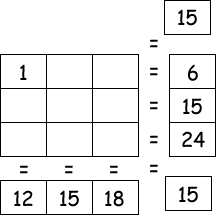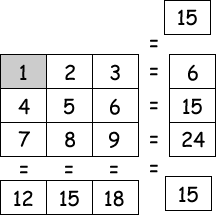Select PagePrintable version

The missing numbers are between 1 & 9. The three numbers in each row add up to the totals on the right, and the columns add up to the totals along the bottom. The sums of the two diagonals are also given. Solve the puzzle by finding all of the missing numbers that satisfy all of the sums as shown.### Solution### Solution explanation

We have been given one value, 1, in the top left corner square, and the totals of each of the horizontal, vertical and diagonal lines.  We need to fill in the blank squares with numbers so that the totals are as shown.

Start by naming each blank square as shown below. (We know A=1, so we can just leave the 1 in there) We now need to find the values of B to I.We can state the values of each letter in 8 equations, like this:

1 + B + C = 6

D + E + F = 15

G + H + I = 24

1 + D + G = 12

B + E + H = 15

C + F + I = 18

1 + E + I = 15

C + E + G = 15

We can also narrow down the possible values for each letter using a table, as shown below (We know that A = 1, so it can’t be 2, or 3, or 4, etc, and similarly, B can’t be 1, and neither can C, etc):
 A 1 B 2 3 4 5 6 7 8 9 C 2 3 4 5 6 7 8 9 D 2 3 4 5 6 7 8 9 E 2 3 4 5 6 7 8 9 F 2 3 4 5 6 7 8 9 G 2 3 4 5 6 7 8 9 H 2 3 4 5 6 7 8 9 I 2 3 4 5 6 7 8 9

Let’s have a look at the first line of the puzzle: 1 + B + C = 6. The value of B + C together must be 5:  B + C = 6 – 1 = 5. This means that B + C could be either 1 + 4 or 2 + 3.  Looking at our grid, we can see that neither B nor C can be 1, as it’s already been taken by A.  This means the second option applies, and B or C is 2, and B or C is 3, and no other letters can be 2 or 3.  We can update the table:

 A 1 B 2 3 C 2 3 D 4 5 6 7 8 9 E 4 5 6 7 8 9 F 4 5 6 7 8 9 G 4 5 6 7 8 9 H 4 5 6 7 8 9 I 4 5 6 7 8 9

Let’s look at the next line: D + E + F = 15. We have quite a few possible combinations here:

9 + 5 + 1

9 + 4 + 2

8 + 6 + 1

8 + 5 + 2

8 + 4 + 3

7 + 6 + 2

7 + 5 + 3

6 + 5 + 4

A quick glance at the table above shows us that we must discount any option that includes a 1, 2 or a 3.  This leaves only one option!  D, E & F can only be 4, 5 or 6.

 A 1 B 2 3 C 2 3 D 4 5 6 E 4 5 6 F 4 5 6 G 7 8 9 H 7 8 9 I 7 8 9

We can update the table to show this, and can now see that G + H + I = 24 can only be 9 + 8 + 7.

Now let’s look at the first vertical line:

1 + D + G = 12

or

D + G = 12 – 1 = 11

Looking at the possible values for D + G:

9 + 2 (won’t work as neither D nor G can be 2)

8 + 3 (won’t work as neither D nor G can be 3)

7 + 4 (this would work if G was 7 and D was 4)

6 + 5 (this won’t work as G can’t be 6 or 5)

So we know that G is 7 and D is 4

 A 1 B 2 3 C 2 3 D 4 E 5 6 F 5 6 G 7 H 8 9 I 8 9

Next, B + E + H = 15 can be solved using the methods shown above, or like this:

 Letters B E H Total All the possible combinations of values are listed – only one gives us the total of 15 2 6 8 16 2 6 9 17 3 6 8 17 3 6 9 18 2 5 8 15 2 5 9 16 3 5 8 16 3 5 9 17

So B = 2, E = 5 and H = 8. Therefore C = 3, F = 6 and I = 9.

 A 1 B 2 C 3 D 4 E 5 F 6 G 7 H 8 I 9

The puzzle is solved!## Feedback

There are more than one way of doing these puzzles and may well be more than one answer.  Please let me and others know what alternatives you find by commenting below.  We also welcome general comments on the subject and any feedback you’d like to give.   If you have a question that need a response from me or you would like to contact me privately, please use the contact form.

## Get more puzzles!

If you’ve enjoyed doing the puzzles, consider ordering the book; 150+ of the best puzzles in a handy pocket sized format. Click here for full details.